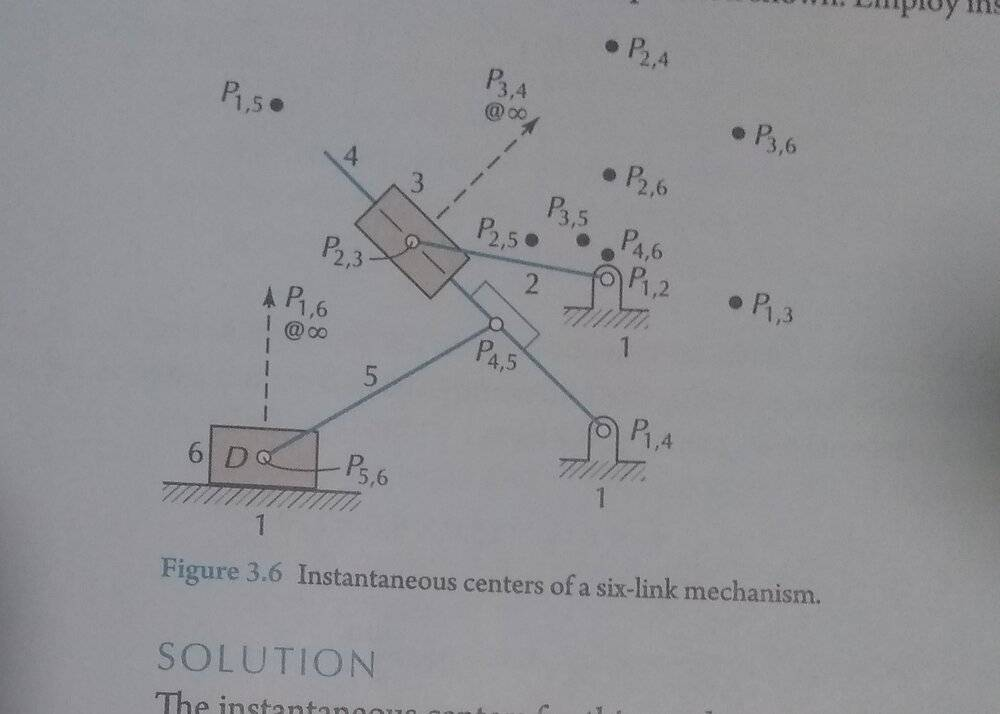# Instantaneous centers of a six bar linkage

Andrew1235
Homework Statement:
Find the velocity of link 6 as a function of the rotational speed of link 2.
Relevant Equations:
The velocity of P 2,6 can be determined by multiplying the angular speed of link 2 by the distance from the ground to P 2,6.I am not sure how to relate the velocities of P 2,6 and slider D. The textbook solution states that they are equal but can someone explain how this can be proven? As far as I understand if P 2,6 is considered as a point on the extended link 2 then it has the same linear velocity as link 6 but why are the velocity directions the same? Is this because P 2,6 can be thought of as moving tangentially on a body and the tangent is horizontal? Also couldn't the point P 2,6 on an extension of link 2 rotate about the slider D?

Last edited:

Homework Helper
Gold Member
2022 Award
You will need to provide a lot of explanation of the diagram.

I take it that the three shaded regions labelled 1 form parts of the same rigid body, and these count as one of the six links.

Labels 2 and 5 are next to what look like links.
Label 4 appears to refer to the line from P1,4 towards P1,5, but if that is all one rigid link then together with links 1 and 2 it forms a triangle, so no movement would be possible.

Label 3 is next to a block. Is that considered a link?

What does the small rectangle around P4,5 represent?

I have been quite unable to make any sense of the Pm,n notation. How does that work?
I do get that the dashed arrows with @∞ mean the label refers to a point at infinity in that direction.

Homework Helper
Gold Member
Homework Statement:: Find the velocity of link 6 as a function of the rotational speed of link 2.
Relevant Equations:: The velocity of P 2,6 can be determined by multiplying the angular speed of link 2 by the distance from the ground to P 2,6.
I am not sure how to relate the velocities of P 2,6 and slider D.
Welcome, Andrew!Do you still need help with this problem?